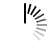# How to Find HCF or GCD using Python?

How to Find HCF or GCD using Python?

Highest Common Factor or Greatest Common Divisor of two or more integers is the largest positive integer that evenly divides the numbers without a remainder. For example, the GCD of 8 and 12 is 4.

```x = int(input("Enter first number: "))
y = int(input("Enter second number: "))
if x > y:
smaller = y
else:
smaller = x
for i in range(1,smaller + 1):
if((x % i == 0) and (y % i == 0)):
hcf = i

print("The H.C.F. of", x,"and", x,"is", hcf)

```